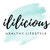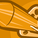# QlikView App Dev

Discussion Board for collaboration related to QlikView App Development.

Announcements
Support Case Portal has moved to Qlik Community! Read the FAQs to start exploring Support resources.
cancel
Showing results for
Did you mean:Contributor III

## Range Expression for Multibox

Hi,

im trying to put range as below

'=if(A>=90 and A<=50,'Mid',if(A>=90,'Hi',if(A<50,'Low')))

but the only expression i get for drop down is Low

I with to have it Hi Mid and Low

This is for expression of Multibox

here is the excel

appreciate if you can copy paste the expression here

Labels (1)
• ### range expression

1 Solution

Accepted SolutionsMVP

May be alternative? 🙂

=if(A>=0.5 and A<=0.9, 'Mid', if(A>0.9, 'Hi', if(A<0.5, 'Low')))

Before develop something, think If placed (The Right information | To the right people | At the Right time | In the Right place | With the Right context)
5 RepliesContributor III
Author

Due to numbering system in my excel,

=if(A>=0.9 and A<=0.5,'Mid',if(A>0.9,'Hi',if(A<0.5,'Low')))

if have succeed to produce Hi and Low but not Mid.

im not sure what wrong with that rangeContributor III
its because A value is not number , it is read as 100% or 50%. either use subfield function in script or use below expression instead

and most importantly delete the g53 column in your attached excel (=if(A>=90 and A<=50,'Mid',if(A>=90,'Hi',if(A<50,'Low'))) ).

The Logic for mid is wrong i.e a>=90 and a<=50 ( there is no number greater than 90 and lesser than 50)
Please find correct expression and corrected excel file .

=if(CEil(num(subfield(A,'%',1)*100)) <=90 and ceil(num(subfield(A,'%',1)*100))>=50,'Mid',if(ceil(num(subfield(A,'%',1)*100)) >=90,'High',if(ceil(num(subfield(A,'%',1)*100)) <50,'Low')))MVP

May be alternative? 🙂

=if(A>=0.5 and A<=0.9, 'Mid', if(A>0.9, 'Hi', if(A<0.5, 'Low')))

Before develop something, think If placed (The Right information | To the right people | At the Right time | In the Right place | With the Right context)Contributor III

please find the corrected excel file and my output attached.Contributor III
Author
thank you so much for the corrected excel. i will use thisCommunity Browser